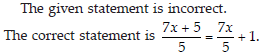Factorisation (Mathematics) Class 8 - NCERT Questions
Q 1.

Find the common factors of the given terms.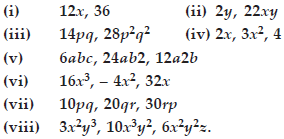SOLUTION: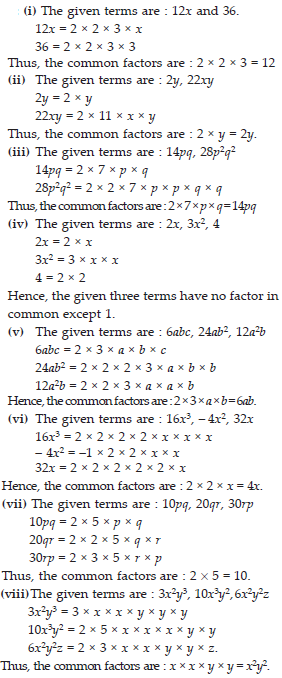.

Q 2.

Factorise the following expressions.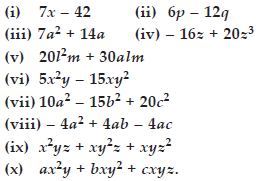SOLUTION: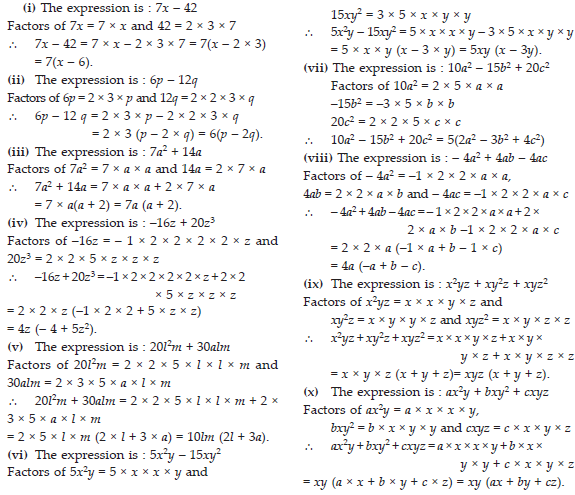Q 3.

Factorise.
(i) x2 + xy + 8x + 8y
(ii) 15xy – 6x + 5y – 2
(iii) ax + bx – ay – by
(iv) 15pq + 15 + 9q + 25p
(v) z – 7 + 7xy – xyz.

SOLUTION: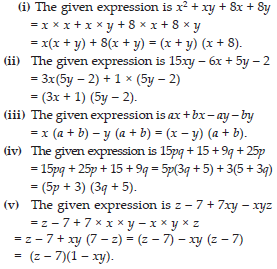Q 4.

Factorise the following expressions.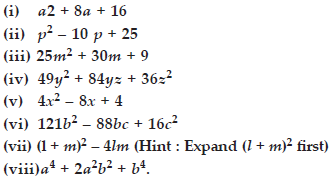SOLUTION: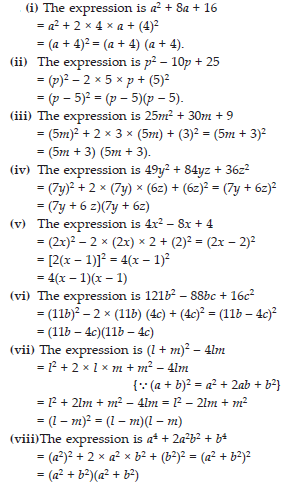Q 5.

Factorise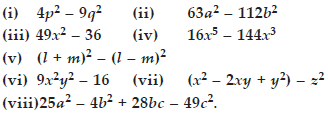SOLUTION: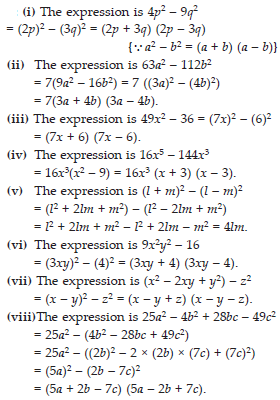Q 6.

Factorise the expressions.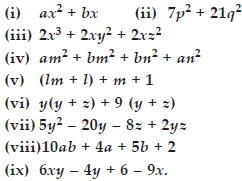SOLUTION: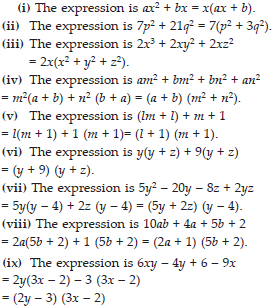Q 7.

Factorise.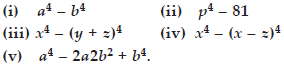SOLUTION: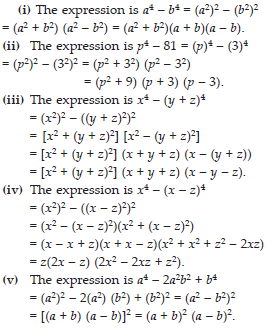Q 8.

Factorise the following expressions.
(i) p2 + 6p + 8
(ii) q2 – 10q + 21
(iii) p2 + 6p – 16.

SOLUTION: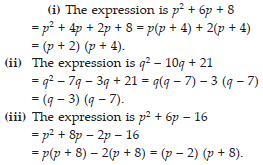Q 9.

Carry out the following divisions.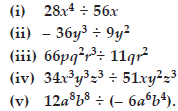SOLUTION: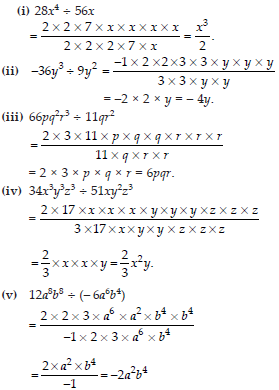Q 10.

Divide the given polynomials by the given monomial.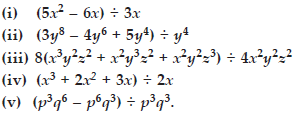SOLUTION: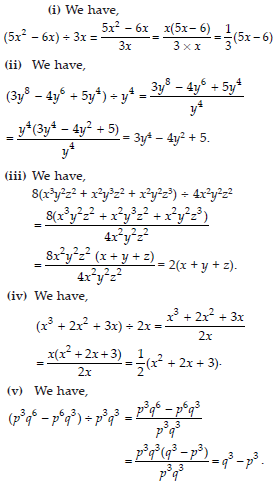Q 11.

Work out the following divisions.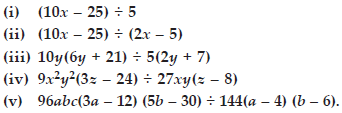SOLUTION: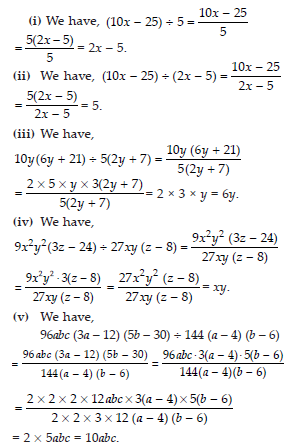Q 12.

Divide as directed.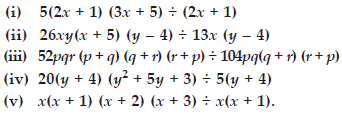SOLUTION: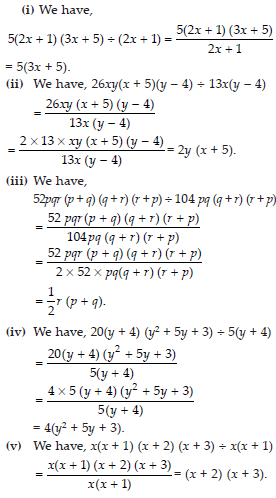Q 13.

Factorise the expressions and divide them as directed.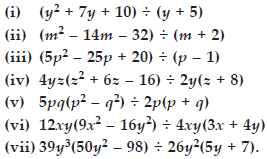SOLUTION: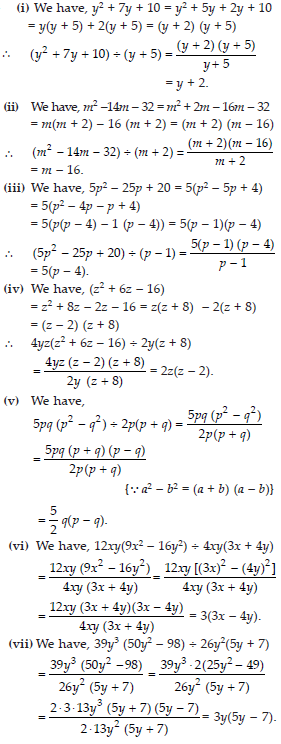Q 14.

Find and correct the errors in the following mathematical statements.
4(x – 5) = 4x – 5

SOLUTION:

The given statement is incorrect
The correct statement is 4(x – 5) = 4x – 20.

Q 15.

x(3x + 2) = 3x2 + 2

SOLUTION:

The given statement is incorrect.
The correct statement is x(3x + 2) = 3x2 + 2x.

Q 16.

2x + 3y = 5xy

SOLUTION:

The given statement is incorrect.
The correct statement is 2x + 3y = 2x + 3y.

Q 17.

x + 2x + 3x = 5x

SOLUTION:

The given statement is incorrect.
The correct statement is x + 2x + 3x = 6x.

Q 18.

5y + 2y + y – 7y = 0

SOLUTION:

The given statement is incorrect.
The correct statement is 5y + 2y + y – 7y = y.

Q 19.

3x + 2x = 5x2

SOLUTION:

The given statement is incorrect.
The correct statement is 3x + 2x = 5x.

Q 20.

(2x)2 + 4(2x) + 7 = 2x2 + 8x + 7

SOLUTION:

The given statement is incorrect.
The correct statement is (2x)2 + 4(2x) + 7
= 4x2 + 8x + 7.

Q 21.

(2x)2 + 5x = 4x + 5x = 9x

SOLUTION:

The given statement is incorrect.
The correct statement is (2x)2 + 5x = 4x2 + 5x.

Q 22.

(3x + 2)2 = 3x2 + 6x + 4

SOLUTION:

The given statement is incorrect.
The correct statement is (3x + 2)2 = (3x)2 + 2(3x)(2) + 22 = 9x2 + 12x + 4.

Q 23.

Substituting x = –3 in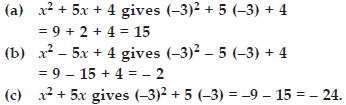SOLUTION: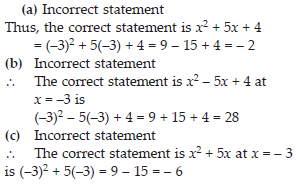Q 24.

(y – 3)2 = y2 – 9

SOLUTION: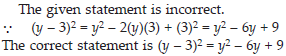Q 25.

(z + 5)2 = z2 + 25

SOLUTION: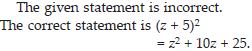Q 26.

(2a + 3b) (a – b) = 2a2 – 3b2

SOLUTION: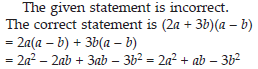Q 27.

(a + 4) (a + 2) = a2 + 8

SOLUTION: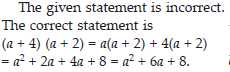Q 28.

(a – 4) (a – 2) = a2 – 8

SOLUTION: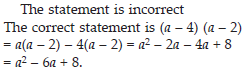Q 29.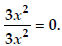SOLUTION: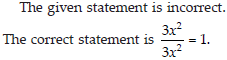Q 30.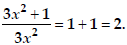SOLUTION: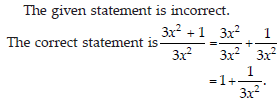Q 31.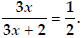SOLUTION: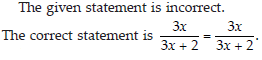Q 32.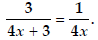SOLUTION: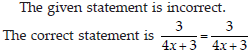Q 33.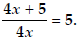SOLUTION: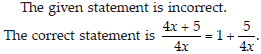Q 34.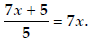SOLUTION: